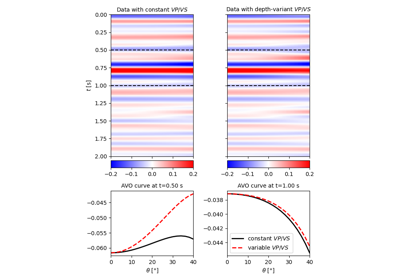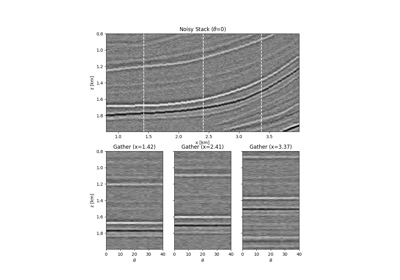# pylops.avo.prestack.PrestackLinearModelling¶

pylops.avo.prestack.PrestackLinearModelling(wav, theta, vsvp=0.5, nt0=1, spatdims=None, linearization='akirich', explicit=False, kind='centered')[source]

Pre-stack linearized seismic modelling operator.

Create operator to be applied to elastic property profiles for generation of band-limited seismic angle gathers from a linearized version of the Zoeppritz equation. The input model must be arranged in a vector of size $$n_m \times n_{t0} (\times n_x \times n_y)$$ for explicit=True and $$n_{t0} \times n_m (\times n_x \times n_y)$$ for explicit=False. Similarly the output data is arranged in a vector of size $$n_{theta} \times n_{t0} (\times n_x \times n_y)$$ for explicit=True and $$n_{t0} \times n_{theta} (\times n_x \times n_y)$$ for explicit=False.

Parameters: wav : np.ndarray Wavelet in time domain (must had odd number of elements and centered to zero). Note that the dtype of this variable will define that of the operator theta : np.ndarray Incident angles in degrees. Must have same dtype of wav (or it will be automatically casted to it) vsvp : float or np.ndarray VS/VP ratio (constant or time/depth variant) nt0 : int, optional number of samples (if vsvp is a scalar) spatdims : int or tuple, optional Number of samples along spatial axis (or axes) (None if only one dimension is available) linearization : str or func, optional choice of linearization, akirich: Aki-Richards, fatti: Fatti, ps: PS or any function on the form of pylops.avo.avo.akirichards explicit : bool, optional Create a chained linear operator (False, preferred for large data) or a MatrixMult linear operator with dense matrix (True, preferred for small data) kind : str, optional Derivative kind (forward or centered). Preop : LinearOperator pre-stack modelling operator. NotImplementedError If linearization is not an implemented linearization NotImplementedError If kind is not forward nor centered

Notes

Pre-stack seismic modelling is the process of constructing seismic pre-stack data from three (or two) profiles of elastic parameters in time (or depth) domain. This can be easily achieved using the following forward model:

$d(t, \theta) = w(t) * \sum_{i=1}^{n_m} G_i(t, \theta) m_i(t)$

where $$w(t)$$ is the time domain seismic wavelet. In compact form:

$\mathbf{d}= \mathbf{G} \mathbf{m}$

On the other hand, pre-stack inversion aims at recovering the different profiles of elastic properties from the band-limited seismic pre-stack data.

## Examples using pylops.avo.prestack.PrestackLinearModelling¶Pre-stack modelling08. Pre-stack (AVO) inversion